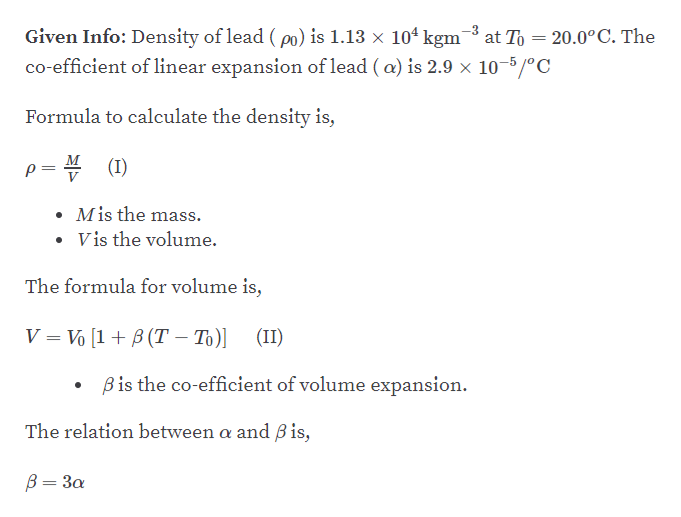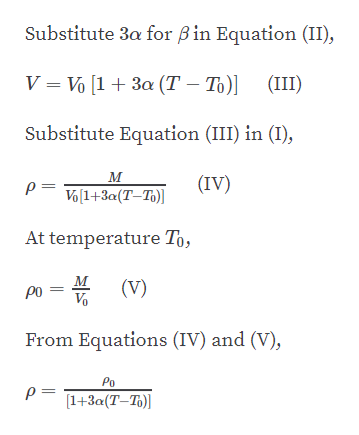# The density of lead is 1.13 x 104 kg/m3 at 20.0°C. Find its density at 105°C.

Question
1 views

The density of lead is 1.13 x 104 kg/m3 at 20.0°C. Find its density at 105°C.

check_circle

Step 1help_outlineImage Transcriptionclose-3 at To = 20.0°C. The Given Info: Density of lead ( po) is 1.13 × 104 kgm co-efficient of linear expansion of lead ( a) is 2.9 × 10-5/°C Formula to calculate the density is, p= 4 (1) • Mis the mass. V is the volume. The formula for volume is, V = Vo [1 + B (T – To)] (II) Bis the co-efficient of volume expansion. The relation between a and Bis, B= 3a fullscreen
Step 2help_outlineImage TranscriptioncloseSubstitute 3a for B in Equation (II), V = Vo [1+ 3a (T – T)] (III) Substitute Equation (III) in (I), м (IV) p= Vo(1+3a(T–To)] At temperature To, м (V) Vo po From Equations (IV) and (V), Po [1+3a(T-To)] || fullscreen

### Want to see the full answer?

See Solution

#### Want to see this answer and more?

Solutions are written by subject experts who are available 24/7. Questions are typically answered within 1 hour.*

See Solution
*Response times may vary by subject and question.
Tagged in

### Other